StatLect

# Boosted linear regression

This lecture introduces a method to train linear regression modelswhere the inputis arow vector, the parameteris avector of regression coefficients and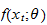is the prediction of the output.

The method is called boosting, and a linear regression model trained with this method is called boosted linear regression.

We are going to assume that both the outputand the entries of the input vectorhave zero mean. In other words, we assume that all the variables have been demeaned (centered) before training the linear regression model.## Algorithm

Boosting is an iterative procedure that yields a sequence of increasingly complex regression models.

We start from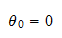Then, at each iteration, we perform the following steps:

1. we compute the regression residuals from the previous iteration: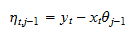2. we find the input variable that has the highest correlation (in absolute value) with the residuals (on the training sample);

3. we estimate by ordinary least squares (on the training sample) the coefficientof the uni-variate regression of the residuals on the chosen variable (suppose it is the-th);

4. we set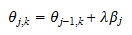whereis the learning rate (usually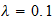); a learning rate less than 1 is used so as to have a gradual increase in complexity and overfitting; all the other entries ofare left unchanged;

5. we compute the mean squared error (MSE) of the regression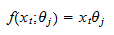on the validation sample;

6. if the MSE has not been decreasing for a pre-set number of iterations, we stop the algorithm.

The boosted regression model, that we use to make predictions, is the most complex one, produced in the last boosting round (iteration of the algorithm).

## Rationale

Boosting usually works very well and yields highly accurate predictive models.

Why? Basically because it is able to reduce a regression problem which is usually high-dimensional and plagued by the curse of dimensionality, to a sequence of uni-dimensional problems that can be solved with high precision.

## Early stopping

The stopping rule in step 6 of the algorithm is called early stopping.

It is a rule used in many iterative machine learning algorithms.

Roughly speaking, we gradually increase model complexity until the performance of the model on the validation sample starts to degrade.

Early stopping is extremely important and is one of the ingredients that explain the good forecasting performance of many machine learning models.

## Example: inflation data set

In our example, we continue to use the same inflation data set used previously.

### Import the data and use scikit-learn to split into train-val-test (60-20-20)

We first import the data and split it into train-val-test.

# Import the packages used to load and manipulate the data
import numpy as np # Numpy is a Matlab-like package for array manipulation and linear algebra
import pandas as pd # Pandas is a data-analysis and table-manipulation tool

# Import the function that performs sample splits from scikit-learn
from sklearn.model_selection import train_test_split

try:
except:
y = y.values # Transform y into a numpy array

# Print some information about the output variable
print('Class and dimension of output variable:')
print(type(y))
print(y.shape)

# Load the input variables with pandas
try:
except:
x = x.values

# Print some information about the input variables
print('Class and dimension of input variables:')
print(type(x))
print(x.shape)

# Create the training sample
x_train, x_val_test, y_train, y_val_test
= train_test_split(x, y, test_size=0.4, random_state=1)

# Split the remaining observations into validation and test
x_val, x_test, y_val, y_test
= train_test_split(x_val_test, y_val_test, test_size=0.5, random_state=1)

# Print the numerosities of the three samples
print('Numerosities of training, validation and test samples:')
print(x_train.shape, x_val.shape, x_test.shape)

The output is:

Class and dimension of output variable:
class 'numpy.ndarray'
(270, 1)
Class and dimension of input variables:
class 'numpy.ndarray'
(270, 113)
Numerosities of training, validation and test samples:
162 54 54

### Create a boosted linear regression class

We create our own class for training boosted linear regression models.

# Import package used to make copies of objects
from copy import deepcopy

# Our boosted linear regression (blr) class will implement 3 methods
# (constructor, fit, and predict), as previously seen in scikit-learn
class blr:
def __init__(self, learning_rate, max_iter, early_stopping):
self.lr = learning_rate
self.max_iter = max_iter
self.early = early_stopping
self.y_mean = 0
self.y_std = 1
self.x_mean = 0
self.x_std = 1
self.theta = 0
self.mses = []

def fit(self, x_train_0, y_train_0, x_val_0, y_val_0):
# Make copies of data to avoid over-writing original dataset
x_train = deepcopy(x_train_0)
y_train = deepcopy(y_train_0)
x_val = deepcopy(x_val_0)
y_val = deepcopy(y_val_0)

# De-mean the output variable
self.y_mean = np.mean(y_train)
y_train -= self.y_mean
y_val -= self.y_mean

# Standardize the output variable
self.y_std = np.std(y_train)
y_train /= self.y_std
y_val /= self.y_std

# De-mean the input variables
self.x_mean = np.mean(x_train, axis=0, keepdims=True)
x_train -= self.x_mean
x_val -= self.x_mean

# Standardize the input variables
self.x_std = np.std(x_train, axis=0, keepdims=True)
x_train /= self.x_std
x_val /= self.x_std

# Initialize counters (total boosting iterations and unproductive iterations)
current_iter = 0
no_improvement = 0

# The starting model has all coefficients equal to zero and predicts a constant zero output
self.theta = np.zeros((x_train.shape, 1))
y_train_pred = 0 * y_train
y_val_pred = 0 * y_val
eta = y_train - y_train_pred
mses = [np.var(y_val - y_val_pred)]

# Boosting iterations
while no_improvement < self.early and current_iter < self.max_iter:
current_iter += 1
corr_coeffs = np.mean(x_train * eta, axis=0) # Correlations (equal to betas) beteen residual and inputs
index_best = np.argmax(np.abs(corr_coeffs)) # Choose variable that has maximum correlation with residual
self.theta[index_best] += self.lr * corr_coeffs[index_best] # Parameter update
y_train_pred += self.lr * corr_coeffs[index_best] * x_train[:, [index_best]] # Prediction update
eta = y_train - y_train_pred # Residuals update
y_val_pred += self.lr * corr_coeffs[index_best] * x_val[:, [index_best]] # Validation prediction update
mses.append(np.var(y_val - y_val_pred)) # New validation MSE
if mses[-1] > np.min(mses[0:-1]): # Stopping criterion to avoid over-fitting
no_improvement += 1
else:
no_improvement = 0

# Final output message
print('Boosting stopped after ' + str(current_iter) + ' iterations')

def predict(self, x_test_0):
# Make copies of the data to avoid over-writing original dataset
x_test = deepcopy(x_test_0)

# De-mean input variables using means on training sample
x_test = x_test - self.x_mean

# Standardize output variables using standard deviations on training sample
x_test = x_test / self.x_std

# Return prediction
return self.y_mean + self.y_std * np.dot(x_test,self.theta)

### Train the boosted linear regression model

We train the boosted regression model with all the 113 input variables.

# Import model-evaluation metrics from scikit-learn
from sklearn.metrics import mean_squared_error, r2_score

# Create a boosted linear regression object
lr = blr(0.1, 10000, 20)

# Train the model
lr.fit(x_train, y_train, x_val, y_val)

# Make predictions on the train, validation and test sets
y_train_pred = lr.predict(x_train)
y_val_pred = lr.predict(x_val)
y_test_pred = lr.predict(x_test)

# Print empirical risk on all sets
print('MSE on training set:')
print(mean_squared_error(y_train, y_train_pred))
print('MSE on validation set:')
print(mean_squared_error(y_val, y_val_pred))
print('MSE on test set:')
print(mean_squared_error(y_test, y_test_pred))
print('')

# Print R squared on all sets
print('R squared on training set:')
print(r2_score(y_train, y_train_pred))
print('R squared on validation set:')
print(r2_score(y_val, y_val_pred))
print('R squared on test set:')
print(r2_score(y_test, y_test_pred))

The output is:

Boosting stopped after 181 iterations
MSE on training set:
0.03676763521269099
MSE on validation set:
0.08231588238762148
MSE on test set:
0.09441771372808147

R squared on training set:
0.7661747762416133
R squared on validation set:
0.6517679287094578
R squared on test set:
0.5446686738671733

This is the best result thus far, better than both 1) selection of the best model among a set of randomly generated ones and 2) selection of a regularized regression model.

Why? Not only we minimized overfitting on the validation set because we basically used it to choose a single parameter (number of boosting rounds), but we also managed to reduce overfitting on the training set by using a smart training strategy (set only a single parameter at a time).